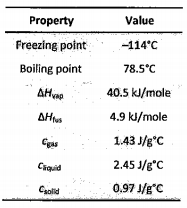# Problem: From the data below, calculate the heat (kJ) involved in the transformation of 0.450 mol of ethanol (CH3CH2OH) gas at 275°C and 1 atm to solid ethanol at -116°C.

###### FREE Expert Solution

We’re being asked to calculate the amount of energy released when 0.450 moles of ethanol gas is cooled from 275 ˚C to -116.0 ˚C (solid ethanol).

Note that cooling and freezing is an exothermic process, which means q is negative (–)

There are two heats involved in this problem:

1. q1 which is the heat in cooling 0.450 moles of gaseous ethanol from 275 ˚C to 78.5 ˚C

2. q2 which is the heat in condensing 0.450 moles of H2O at 78.5 ˚C

3. q3 which is the heat in cooling 0.450 moles of liquid ethanol from 78.5 ˚C to -114˚C

4. q3 which is the heat in freezing 0.450 moles of H2O at -114˚C

5. q3 which is the heat in cooling 0.450 moles of solid ethanol from -114˚C to -116 ˚C

We need to solve for each heat individually then add them together to get the final answer.

91% (56 ratings)###### Problem Details

From the data below, calculate the heat (kJ) involved in the transformation of 0.450 mol of ethanol (CH3CH2OH) gas at 275°C and 1 atm to solid ethanol at -116°C.Frequently Asked Questions

What scientific concept do you need to know in order to solve this problem?

Our tutors have indicated that to solve this problem you will need to apply the Heating and Cooling Curves concept. You can view video lessons to learn Heating and Cooling Curves. Or if you need more Heating and Cooling Curves practice, you can also practice Heating and Cooling Curves practice problems.

What is the difficulty of this problem?

Our tutors rated the difficulty ofFrom the data below, calculate the heat (kJ) involved in the...as high difficulty.